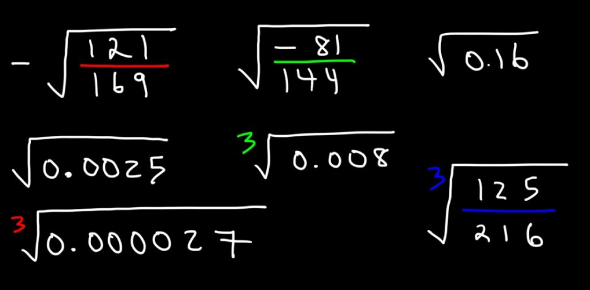Square And Cube Roots Quiz Questions

13 Questions | Total Attempts: 4907SettingsTest your knowledge on this square and cube roots quiz to see how you do and compare your score to others.

• 1.
First, decide if the following number is a perfect square. If it IS, then identify the square root for the expression. √16
• A.

No, it is not a perfect square.

• B.

Yes, it is a perfect square, and the square root is 2.

• C.

Yes, it is a perfect square, and the square root is 4.

• D.

Yes, it is a perfect square, and the square root is 8.

• 2.
First, decide if the following number is a perfect square. If it IS, then identify the square root for the expression. √64
• A.

No, it is not a perfect square.

• B.

Yes, it is a perfect square, and the square root is 4.

• C.

Yes, it is a perfect square, and the square root is 8.

• D.

Yes, it is a perfect square, and the square root is 32.

• 3.
First, decide if the following number is a perfect square. If it IS, then identify the square root for the expression. √100
• A.

No, it is not a perfect square.

• B.

Yes, it is a perfect square, and the square root is 5.

• C.

Yes, it is a perfect square, and the square root is 1.

• D.

Yes, it is a perfect square, and the square root is 10.

• 4.
First, decide if the following number is a perfect square. If it IS, then identify the square root for the expression. √121
• A.

No, it is not a perfect square.

• B.

Yes, it is a perfect square, and the square root is 11.

• C.

Yes, it is a perfect square, and the square root is 12.

• D.

Yes, it is a perfect square, and the square root is 13.

• 5.
First, decide if the following number is a perfect square. If it IS, then identify the square root for the expression. √0
• A.

No, it is not a perfect square.

• B.

Yes, it is a perfect square, and the square root is 1.

• C.

Yes, it is a perfect square, and the square root is 0.

• D.

Yes, it is a perfect square, and the square root is 10.

• 6.
√19 falls between the square root of which 2 numbers? (The square root chart that you got in class will really help to answer this question!)
• A.

3 and 4, and it is approximately 3.7

• B.

4 and 5, and it is approximately 4.4

• C.

5 and 6, and it is approximately 5.3

• D.

2 and 3, and it is approximately 2.4

• 7.
√40 falls between the square root of which 2 numbers? (The square root chart that you got in class will really help to answer this question!)
• A.

4 and 5, and it is approximately 4.2

• B.

5 and 6, and it is approximately 5.5

• C.

6 and 7, and it is approximately 6.3

• D.

3 and 4, and it is approximately 3.3

• 8.
√75 falls between the square root of which 2 numbers? (The square root chart that you got in class will really help to answer this question!)
• A.

6 and 7, and it is approximately 6.7

• B.

7 and 8, and it is approximately 7.4

• C.

8 and 9, and it is approximately 8.7

• D.

5 and 6, and it is approximately 5.1

• 9.
√111 falls between the square root of which 2 numbers? (The square root chart that you got in class will really help to answer this question!)
• A.

8 and 9, and it is approximately 8.6

• B.

9 and 10, and it is approximately 9.7

• C.

10 and 11, and it is approximately 10.5

• D.

11 and 12, and it is approximately 11.1

• 10.
Emma's bedroom is shaped like a square.  What are the dimensions of the room if the area of the floor is 196 square feet?
• A.

49 ft x 49 ft

• B.

7 ft x 7 ft

• C.

14 ft x 14 ft

• D.

4 ft x 49 ft

• 11.
What is the square of 7?
• A.

49

• B.

14

• C.

28

• D.

3.5

• 12.
If the volume of a cube is 64 inches. What is the length of edges?
• A.

16

• B.

4

• C.

21.3

• 13.
If the volume of a cube is 512 feet. What is the length of its edges?
• A.

8 feet

• B.

128 feet

• C.

246 feet

Related TopicsBack to top